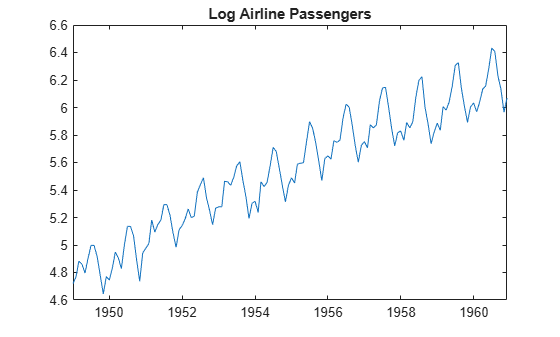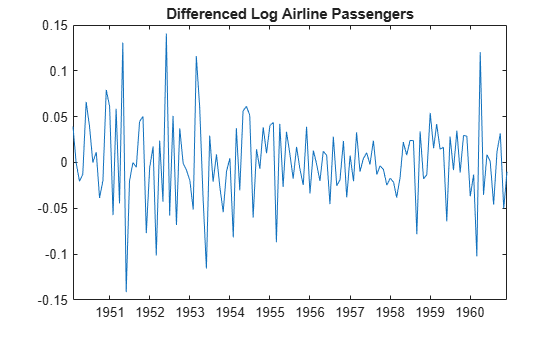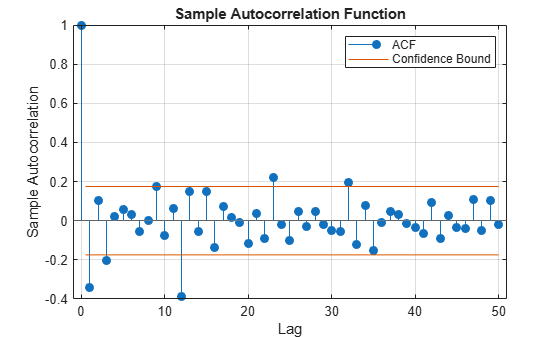# Create Multiplicative Seasonal ARIMA Model for Time Series Data

This example shows how to specify a seasonal ARIMA model using `arima`. The time series is monthly international airline passenger numbers from 1949 to 1960.

### Load the airline passenger data.

Load the airline data set, and then plot the natural log of the monthly passenger totals.

```load Data_Airline y = log(DataTimeTable.PSSG); T = length(y); figure plot(DataTimeTable.Time,y) title("Log Airline Passengers")```The series appears nonstationary, with a linear trend and seasonal periodicity.

### Plot the seasonally integrated series.

Calculate the differenced series, $\left(1-L\right)\left(1-{L}^{12}\right){y}_{t}$, where ${y}_{t}$ is the original log-transformed data. Plot the differenced series.

```A1 = LagOp({1,-1},Lags=[0 1]); A12 = LagOp({1,-1},Lags=[0 12]); dY = filter(A1*A12,y); dT = T - length(dY); figure plot(DataTimeTable.Time((dT+1):T),dY) title("Differenced Log Airline Passengers")```The differenced series appears stationary.

### Plot the sample autocorrelation function (ACF).

```figure autocorr(dY,NumLags=50)```The sample ACF of the differenced series shows significant autocorrelation at lags that are multiples of 12. There is also potentially significant autocorrelation at smaller lags.

### Specify a seasonal ARIMA model.

Box, Jenkins, and Reinsel suggest the multiplicative seasonal model,

`$\left(1-L\right)\left(1-{L}^{12}\right){y}_{t}=\left(1-{\theta }_{1}L\right)\left(1-{\Theta }_{12}{L}^{12}\right){\epsilon }_{t},$`

for this data set .

Specify this model.

```Mdl = arima(Constant=0,D=1,Seasonality=12, ... MALags=1,SMALags=12)```
```Mdl = arima with properties: Description: "ARIMA(0,1,1) Model Seasonally Integrated with Seasonal MA(12) (Gaussian Distribution)" SeriesName: "Y" Distribution: Name = "Gaussian" P: 13 D: 1 Q: 13 Constant: 0 AR: {} SAR: {} MA: {NaN} at lag  SMA: {NaN} at lag  Seasonality: 12 Beta: [1×0] Variance: NaN ```

The property `P` is equal to `13`, corresponding to the sum of the nonseasonal and seasonal differencing degrees (1 + 12). The property `Q` is also equal to `13`, corresponding to the sum of the degrees of the nonseasonal and seasonal MA polynomials (1 + 12). Parameters that need to be estimated have value `NaN`.

 Box, George E. P., Gwilym M. Jenkins, and Gregory C. Reinsel. Time Series Analysis: Forecasting and Control. 3rd ed. Englewood Cliffs, NJ: Prentice Hall, 1994.